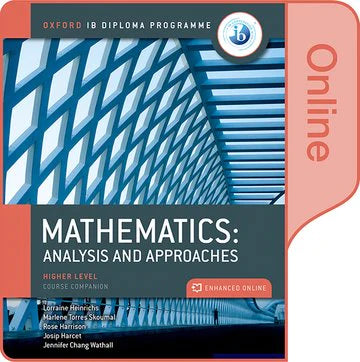# IB Mathematics: analysis and approaches, HL Course Companion

Regular price \$115.00
Sale price \$115.00 Regular price
Featuring a wealth of digital content, this concept-based Print and Enhanced Online Course Book Pack has been developed in cooperation with the IB to provide the most comprehensive support for the new DP Mathematics: analysis and approaches HL syllabus, for first teaching in September 2019.

### Features

• Address all aspects of the new DP Mathematics: analysis and approaches HL syllabus via an Enhanced Online Course Book Pack - made up of one full-colour, print textbook and one online textbook, including extensive teacher notes
• Ensure learners are ready to tackle each topic with targeted 'Prior Knowledge' worksheets, linked to 'Before You Start' summaries and exercises at the start of every chapter
• Deliver in-depth coverage of all topics through clear explanations and worked solutions, animated worked examples, differentiated exercises and worksheets, with answers provided
• Adopt a concept-based approach with conceptual lenses and microconcepts woven into every chapter, plus rich investigations that integrate factual and conceptual questions - leading to meaningful, content-specific conceptual understanding
• Deepen mathematical understanding via inquiry-based tasks that relate to the content of each chapter, 'international mindedness' features, regular links to Theory of Knowledge, and activities that target ATL skills
• Support students' development of a mathematical toolkit, as required by the new syllabus, with modelling and investigation activities presented in each chapter, including prompts for reflection, and suggestions for further study
• Thoroughly prepare students for IB assessment via in-depth coverage of course content, overviews of all requirements, exam-style practice questions and papers, and a full chapter supporting the new mathematical exploration (IA)
• Includes support for the most popular Graphic Display Calculator models
• This Online Course Book will be available on Oxford Education Bookshelf until 2029. Access is facilitated via a unique code, which is sent in the mail. The code must be linked to an email address, creating a user account.
• Access may be transferred once to an additional user.

From patterns to generalizations: sequences and series
1.1: Sequences, series and sigma notation
1.2: Arithmetic and geometric sequences and series
1.3: Proof
1.4: Counting principles and the binomial theorem
Representing relationships: introducing functions
2.1: Functional relationships
2.2: Special functions and their graphs
2.3: Classification of functions
2.4: Operations with functions
2.5: Function transformations
Expanding the number system: complex numbers
3.2: Complex numbers
3.3: Polynomial equations and inequalities
3.4: The fundamental theorem of algebra
3.5: Solving equations and inequalities
3.6: Solving systems of linear equations
Measuring change: differentiation
4.1: Limits, continuity and convergence
4.2: The derivative of a function
4.3: Differentiation rules
4.4: Graphical interpretation of the derivatives
4.5: Applications of differential calculus
4.6: Implicit differentiation and related rates
Analysing data and quantifying randomness: statistics and probability
5.1: Sampling
5.2: Descriptive statistics
5.3: The justification of statistical techniques
5.4: Correlation, causation and linear regression
Relationships in space: geometry and trigonometry
6.1: The properties of 3D space
6.2: Angles of measure

6.3: Ratios and identities
6.4: Trigonometric functions
6.5: Trigonometric equations
Generalizing relationships: exponents, logarithms and integration
7.1: Integration as antidifferentiation and definite integrals
7.2: Exponents and logarithms
7.3: Derivatives of exponential and logarithmic functions; tangents and normals
7.4: Integration techniques
Modelling changes: more calculus
8.1: Areas and volumes
8.2: Kinematics
8.3: Ordinary differential equations (ODEs)
8.4: Limits revisited
Modelling 3D space: vectors
9.1: Geometrical representation of vectors
9.2: Introduction to vector algebra
9.3: Scalar product and its properties
9.4: Vector equations of a line
9.5: Vector product and properties
9.6: Vector equation of a plane
9.7: Lines, planes and angles
9.8: Application of vectors
Equivalent systems of representation: more complex numbers
10.1: Forms of a complex number
10.2: Operations with complex numbers in polar form
10.3: Powers and roots of complex numbers in polar form
Valid comparisons and informed decisions: probability distributions
11.1: Axiomatic probability systems
11.2: Probability distributions
11.3: Continuous random variables
11.4: Binomial distribution
11.5: The normal distribution
Exploration

Author: Marlene Torres Skoumal, Rose Harrison, Josip Harcet, Jennifer Chang Wathall, and Lorraine Heinrichs

Curriculum: IB Diploma Programme International Baccalaureate

Dimension: 10 x 1.3 x 7.7 inches

Format: Paperback

Isbn 10: 0198427166

Language: English

Pages: 832 pages

Release date: 05/21/2019

Series: Oxford IB Diploma Programme IB Mathematics

Year: 2019